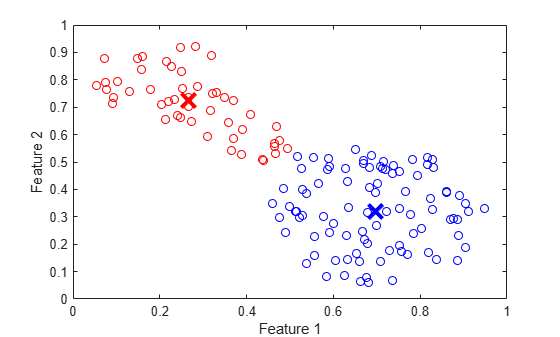# fcm

Fuzzy c-means clustering

## Syntax

``````[centers,U] = fcm(data)``````
``````[centers,U] = fcm(data,options)``````
``````[centers,U,objFunc] = fcm(___)``````

## Description

example

``````[centers,U] = fcm(data)``` performs fuzzy c-means clustering on the data using default options.```

example

``````[centers,U] = fcm(data,options)``` specifies clustering options, including the number of clusters and the clustering exponent.```

example

``````[centers,U,objFunc] = fcm(___)``` returns the objective function values at each optimization iteration for all of the previous syntaxes.```

## Examples

collapse all

Load the data to cluster. Each row of `fcmdata` contains one data point. The two columns of `fcmdata` contain the feature values for each data point.

`load fcmdata.dat`

Specify clustering options using an `fcmOptions` object. For this example, set the number of clusters to 2 and use default values for the other options.

`options = fcmOptions(NumClusters=2);`

Find the cluster centers using fuzzy c-means clustering.

`[centers,U] = fcm(fcmdata,options);`
```Iteration count = 1, obj. fcn = 8.970479 Iteration count = 2, obj. fcn = 7.197402 Iteration count = 3, obj. fcn = 6.325579 Iteration count = 4, obj. fcn = 4.586142 Iteration count = 5, obj. fcn = 3.893114 Iteration count = 6, obj. fcn = 3.810804 Iteration count = 7, obj. fcn = 3.799801 Iteration count = 8, obj. fcn = 3.797862 Iteration count = 9, obj. fcn = 3.797508 Iteration count = 10, obj. fcn = 3.797444 Iteration count = 11, obj. fcn = 3.797432 Iteration count = 12, obj. fcn = 3.797430 Minimum improvement reached. ```

Classify each data point into the cluster with the largest membership value.

```maxU = max(U); index1 = find(U(1,:) == maxU); index2 = find(U(2,:) == maxU);```

Plot the clustered data and cluster centers.

```plot(fcmdata(index1,1),fcmdata(index1,2),"ob") hold on plot(fcmdata(index2,1),fcmdata(index2,2),"or") plot(centers(1,1),centers(1,2),"xb",MarkerSize=15,LineWidth=3) plot(centers(2,1),centers(2,2),"xr",MarkerSize=15,LineWidth=3) xlabel("Feature 1") ylabel("Feature 2") hold off```Create a random data set.

`data = rand(100,2);`

Specify the following FCM clustering options.

• Compute two clusters.

• To increase the amount of fuzzy overlap between the clusters, specify a large fuzzy partition matrix exponent.

• Suppress the command-window display of the objective function values for each iteration.

```options = fcmOptions(... NumClusters=2,... Exponent=3.0,... Verbose=false);```

Cluster the data.

`[centers,U] = fcm(data,options);`

`load clusterDemo.dat`

Configure an options object for computing three clusters and suppress the command-window output of the objective function values. Also, set the clustering termination conditions such that the optimization stops when either of the following occurs:

• The number of iterations reaches a maximum of 50.

• The objective function improves by less than 0.001 between two consecutive iterations.

```options = fcmOptions(... NumClusters=3,... MaxNumIteration=50,... MinImprovement=0.001,... Verbose=false);```

Cluster the data.

`[centers,U,objFun] = fcm(clusterDemo,options);`

The length of the objective function vector is less than 50; therefore the clustering did not reach the maximum number of iterations.

View the final three values of the objective function vector.

`objFun(end-2:end)`
```ans = 3×1 15.4353 15.4306 15.4305 ```

The optimization stopped because the objective function improved by less than 0.001 between the final two iterations.

## Input Arguments

collapse all

Data set to be clustered, specified as a matrix with Nd rows, where Nd is the number of data points. The number of columns in `data` is equal to the data dimensionality, that is, the number of features in each data point.

Clustering options, specified as an `fcmOptions` object.

## Output Arguments

collapse all

Final cluster centers, returned as a matrix with Nc rows containing the coordinates of each cluster center, where Nc is the number of clusters specified using `options.NumClusters`. The number of columns in `centers` is equal to the dimensionality of the data being clustered.

Fuzzy partition matrix, returned as an Nc-by-Nd matrix. Element `U(i,j)` indicates the degree of membership μij of the jth data point in the ith cluster. For a given data point, the sum of the membership values for all clusters is one.

Objective function values for each iteration, returned as a vector.

## Tips

• To generate a fuzzy inference system using FCM clustering, use the `genfis` function. For example, suppose that you cluster your data using the following syntax.

`[centers,U] = fcm(data,fcmOpt);`

The first `M` columns of `data` correspond to input variables and the remaining columns correspond to output variables.

You can generate a fuzzy system using the same training data and FCM clustering configuration. To do so:

1. Configure the clustering options.

```opt = genfisOptions("FCMClustering"); opt.NumClusters = fcmOpt.NumClusters; opt.Exponent = fcmOpt.Exponent; opt.MaxNumIteration = fcmOpt.MaxNumIteration; opt.MinImprovement = fcmOpt.MinImprovement; opt.DistanceMetric = fcmOpt.DistanceMetric; opt.Verbose = fcmOpt.Verbose; ```
2. Extract the input and output variable data.

```inputData = data(:,1:M); outputData = data(:,M+1:end); ```
3. Generate the FIS structure.

`fis = genfis(inputData,outputData,opt);`

The fuzzy system `fis` contains one fuzzy rule for each cluster, and each input and output variable has one membership function per cluster. For more information, see `genfis` and `genfisOptions`.

## Algorithms

FCM is a clustering method that allows each data point to belong to multiple clusters with varying degrees of membership. To configure clustering options, create an `fcmOptions` object.

The FCM algorithm computes cluster centers and membership values to minimize the following objective function.

`${J}_{m}=\sum _{i=1}^{C}\sum _{j=1}^{N}{\mu }_{ij}^{m}{D}_{ij}^{2}$`

Here:

• N is the number of data points.

• C is the number of clusters. To specify this value, use the `NumClusters` option.

• m is fuzzy partition matrix exponent for controlling the degree of fuzzy overlap, with m > 1. Fuzzy overlap refers to how fuzzy the boundaries between clusters are, that is, the number of data points that have significant membership in more than one cluster. To specify the fuzzy partition matrix exponent, use the `Exponent` option.

• Dij is the distance from the jth data point to the ith cluster.

• μij is the degree of membership of the jth data point in the ith cluster. For a given data point, the sum of the membership values for all clusters is one.

The `fcm` function supports two types of FCM clustering: classical FCM and Gustafson-Kessel FCM. These methods differ in the distance metric used for computing Dij. For more information, see Fuzzy Clustering.

 Bezdek, James C. Pattern Recognition with Fuzzy Objective Function Algorithms. Boston, MA: Springer US, 1981. https://doi.org/10.1007/978-1-4757-0450-1.